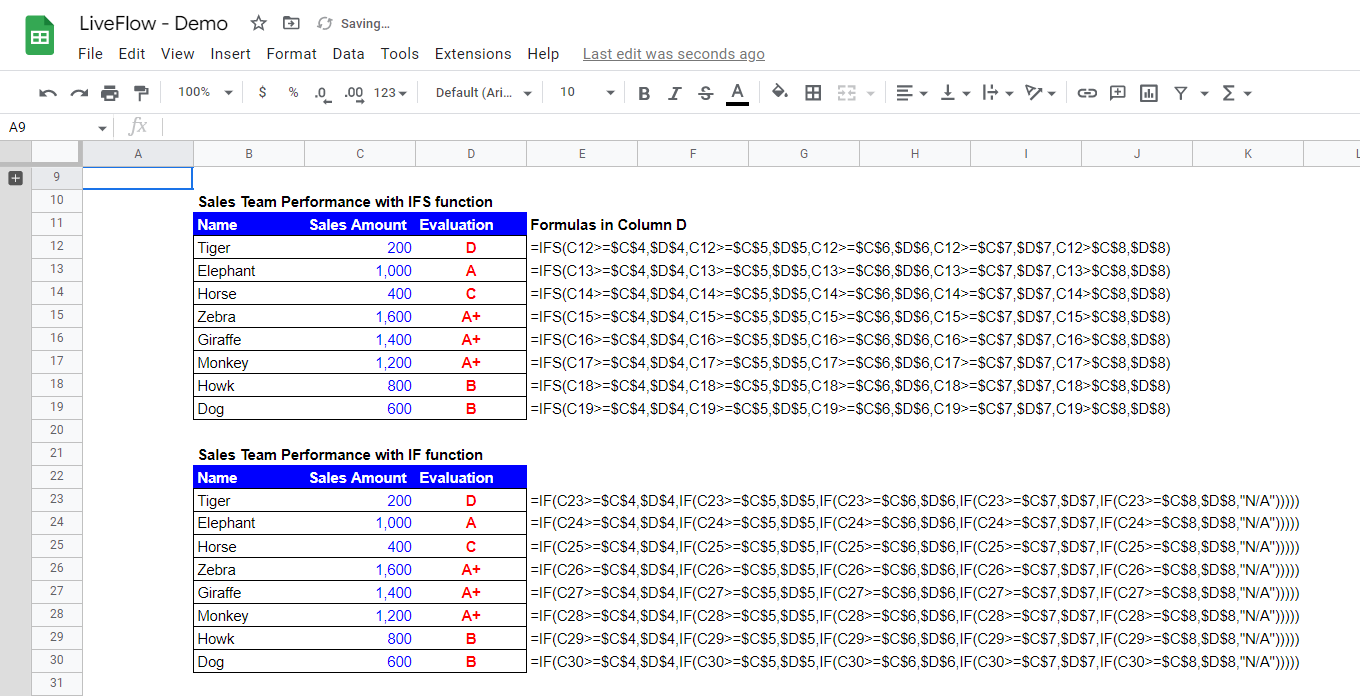# How to Use IFS Function in Google Sheets

In this article, you will learn how to utilize the IFS function in Google Sheets. This function is helpful when you set up multiple conditions and corresponding returns (e.g., giving Grade A through E depending on if a score meets a criterion of each grade)

## How to use the IFS formula in Google Sheets

1. Type “=IFS(” or go to “Insert”“Function”“Logical”“IFS”
2. Insert pairs of a condition and a return (when the condition is met) until you incorporate all conditions
3. Press the “Enter” key

Generic formula

``=IFS(condition1, value1, [condition2, …], [value2, …])``

Condition1: This is a standard to test a given value.

Value1: This is the value returned by the IFS formula when Condition1 is met.

Imagine you are a general manager of a sales team and need to evaluate the performance of each member. Your company has a 5-level grade system for sales performance. The upper table, Evaluation Criteria, in the picture summarizes the grading system.

A tested number is given;
Grade A+ when equal to or more than 1,200;
Grade A when equal to or more than 900;
Grade B when equal to or more than 600;
Grade C when equal to or more than 300, and
Grade D when equal to or more than zero.

In Sales Team Performance with IFS function table, you can see the grades returned by the formulas, and each grade satisfies each criterion. The IFS formulas in Column D next to the Sales Amount test the Sales Amounts and drop them to the right place. For instance, 200 by Tiger is more than 0 but less than 300; Tiger’s Grade is, unfortunately, D.

## What is the difference between IF and IFS in Google Sheets?

Look at the picture below. You can use the IF formula as an alternative to the IFS function by combining the IF formulas repeatedly in one IF formula. However, as you can see, the IFS function is shorter, easy to follow, and more visible, compared to the combination of the IF functions. The repetitive usage of IF formulas tends to look complicated because of many parentheses, and you often get lost while working the formula. We recommend that you use the IFS function when you need to set up a series of criteria and get the values returned according to the requirements.A comparison of the IF function and the IFS function with examples

Learn how to do this step-by-step in the video below 👇

## Need help?

Our team is here to help you any time between 9am and 10pm EST.Email us at: help@liveflow.io